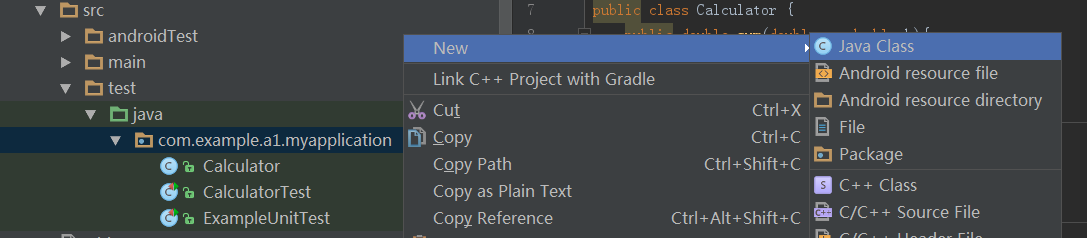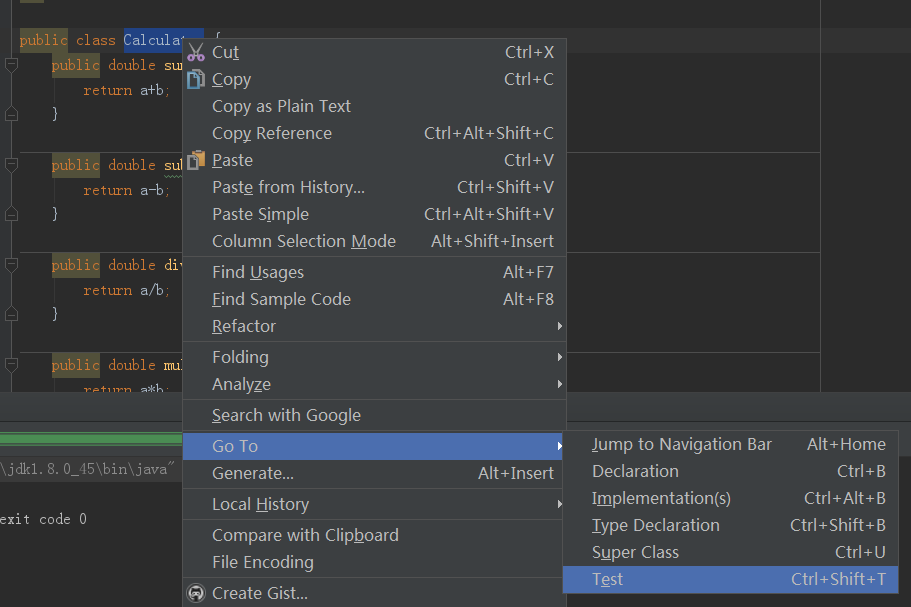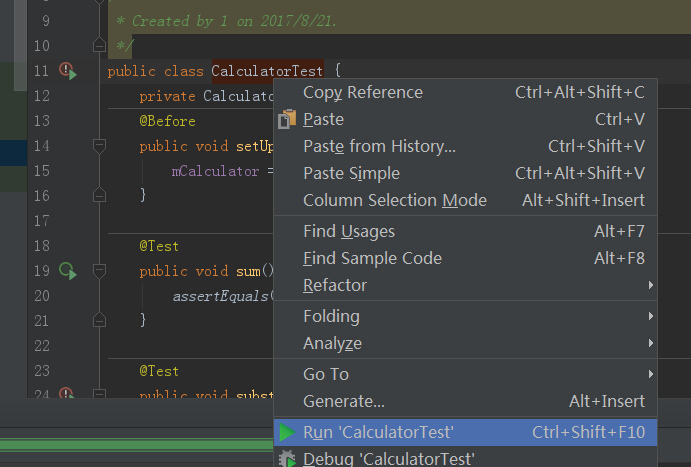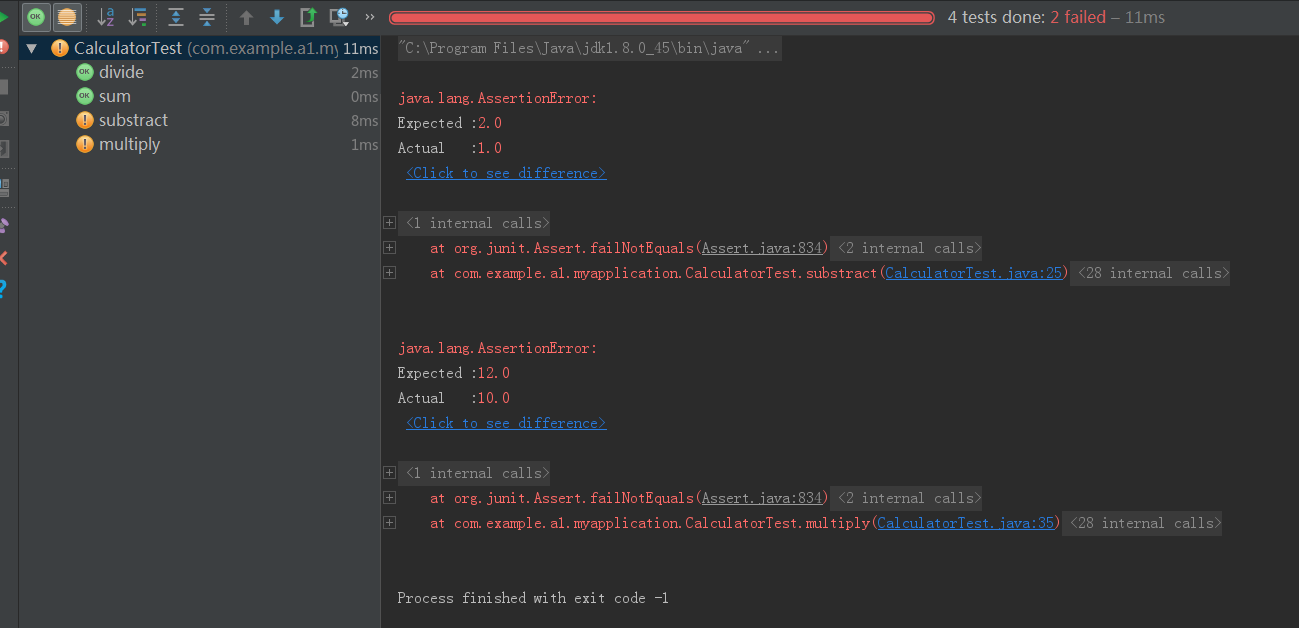# Android Studio中进行单元测试

dependencies {
compile fileTree(include: ['*.jar'], dir: 'libs')
testCompile 'junit:junit:4.12'
}package com.example.a1.myapplication;

/**
* Created by 1 on 2017/8/21.
*/

public class Calculator {
public double sum(double a, double b){
return a+b;
}

public double substract(double a, double b){
return a-b;
}

public double divide(double a, double b){
return a/b;
}

public double multiply(double a, double b){
return a*b;
}
}package com.example.a1.myapplication;

import org.junit.Before;
import org.junit.Test;

import static org.junit.Assert.*;

/**
* Created by 1 on 2017/8/21.
*/
public class CalculatorTest {
private Calculator mCalculator;
@Before
public void setUp() throws Exception {
mCalculator = new Calculator();
}

@Test
public void sum() throws Exception {
assertEquals(6d, mCalculator.sum(1d, 5d), 0);
}

@Test
public void substract() throws Exception {
assertEquals(2d, mCalculator.substract(5d, 4d), 0);
}

@Test
public void divide() throws Exception {
assertEquals(3d, mCalculator.divide(20d, 5d), 1);
}

@Test
public void multiply() throws Exception {
assertEquals(12d, mCalculator.multiply(2d, 5d), 1);
}

}